##1. Asymptotic Triangles

Asymptotic triangle of a hyperbola is a triangle ABC whose sides are the two asymptotes and a tangent of the hyperbola.

All the asymptotic triangles of the hyperbola have the same area E, equal to the product a*b of the axes of the hyperbola. The figure below shows that there is a circle through the focal points of the hyperbola, and points A, C , where the tangent cuts the asymptotes. The three triangles AEC, BCF, FBA are similar, having their angles at B and E equal to the fixed angle FBA (depending on the hyperbola).
Notice a property of the contact point D of the tangent: it is the middle of the segment AC. Notice also that the asymptotic triangle of the conjugate hyperbola has the same area with ABC.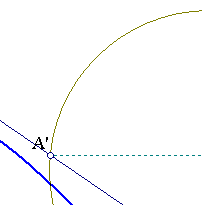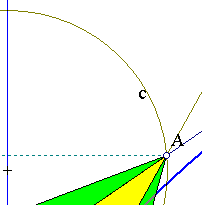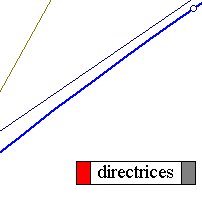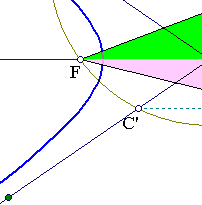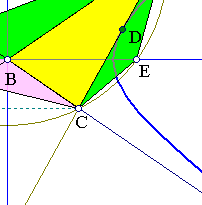##2. Determination of focal points

Knowing an asymptotic triangle ABC of the hyperbola and that its center is at B we can find the whole hyperbola by determining its focal points. In fact construct the bisectors at B and take the relfected {A',C'} of {A,C} at the exterior bisector of B. The trapezium ACC'A' has a circumcircle c which passes through the focal points {E,F} of the hyperbola. The focal points are the intersection points of c with the inner bisector of angle B of the triangle.
The property can be read inversely as a relation between hyperbolas and isosceli trapezia:
Given an isosceles trapezium ACC'A' there is a unique hyperbola having its diagonals as asymptotes and triangle ABC as asymptotic. Its focal points are at the intersections of the circumcircle of the trapezium with the bisector at B... etc. (see also AsymptoticTriangleInv.html ).

Look at the file QuadDivision.html for a nice application of these facts to the problem of dividing a quadrangle in four equal parts.